## Percentage For SBI PO : Set -11

1) The ratio of the number of students studying in schools A,B and C IS 5:6:8.If the number of students is increased by 30%,25% and 25% respectively. What will be the new ratio of the students in schools A, B and C ?

a) 14:15:20

b) 13:15:20

c) 13:14:15

d) 15:17:19

e) None of these

b)

Let the number of students in A, B, C be 5x, 6x & 8x respectively.

New strength = (130 % of 5x), (125 % of 6x) & (125 % of 8x)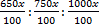= 65:75:100

= 13 : 15 : 20

2) A clerk received an annual salary of Rs.3,660 in the year 1975. This was 20% more than his salary in 1974. What was the salary in 1974?

a) Rs 3,005

b) Rs 3,000

c) Rs 3,500

d) Rs 3,050

e) None of these

d) Rs.3050

Clerk salary in 7975 = Rs. 3660

In 1974, 20% more than his salary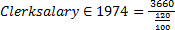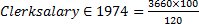= Rs. 3050

3) In an examination paper of five questions, 5% of the conditions answered all of them and 5% answered none. Of the rest, 25% candidates answered only one question and 20% answered 4 questions. If 396 candidates answered either 2 questions or 3 questions, the number of candidates that appeared for the examination was:

a) 850

b) 900

c) 800

d) 1,000

e) none of these

c)

Let total No. of candidates = 1000

So, 1000 – (50+50+900*1/4+900*1/5) =396

495=396

1000=396/495 * 1000

= 800

4) Due to an increase of 20% in the price of eggs, 2 eggs less are available for Rs 24.The present rate of eggs per dozen is :

a) Rs 25.00

b) Rs 26.20

c) Rs 27.80

d) Rs 28.80

e) None of these

d)

20% of 24=4.8

Due to increase, 2 eggs cost Rs.4.8

Rate of eggs per dozen=4.8/2×12=28.8

5) Raj save 10%, after 2 years when his income is increased by 10%, he could save the same money then how much his expenditure increased?

a) 10

b) 20

c) 5

d) 15

e) None

a)

We don’t know the exact income of Raj. We can find the percentage of increase in expenditure as 11.11%

6) Ganesh Monthly income is 4/5th of Sureshs Monthly income The respective ration between sureshs and Sunils monthly income is 13:17. Sunil Spends 12% of his monthly income on food expenses which is Rs. 8160. What is the annual income of Ganesh?

a) Rs. 499100

b) Rs. 492900

c) Rs. 499200

d) Rs. 499300

e) None of these

c)

12% -> 8160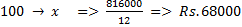Sureshs monthly incomes:-

17 = 68000

13 ->     68000*13/17=520000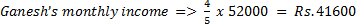his annual income = Rs.(12 x 41600)  Rs.499200

7) Rahim spends 60% of his monthly salary on rent, EMI and miscellaneous expenses in the ratio of 2:1:3. If he spends a total of Rs.16050 on rent and EMI together, how much is his monthly salary?

a) Rs.50300

b) Rs.49600

c) Rs.46750

d) Rs.53500

e) Rs.54500

d)

Rent : EMI : Miscellaneous

2: 1: 3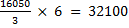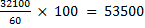8) A man spends 50% of his income in board and lodging, 20% of the remainder in other personal necessities and 25% of the rest in charity, find his income, if he is left with Rs. 4200.

a) Rs. 14000

b) Rs. 8000

c) Rs. 12000

d) Rs. 18000

e) None of these

a) Total income =x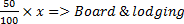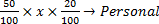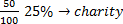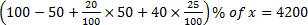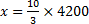x=14000

9) A sold an article at 10 per cent loss on the cost price. He had bought it at a discount of 20 per cent on the labelled price. What would have been the percentage loss had he bought it at the labelled price?

a) 34

b) 18

d) 28

e) 16

d)

Labelled Price 100 %

He Bought at 20% of Discount = 80%

Sold at 10% Loss = 72%

Loss From Labelled Price = 100-72=28%

10) Ashmit can solve 80% of the problem given in a book and Amisha can solve 70%. What is the probability that at least one of them will solve a problem, selected at random from the book?

a) 0.60

b) 0.06

c) 0.94

d) 0.56

e) 0.53

c) 0.94

Probability that Ashmit and Amisha can solve

= 0.8 x 0.7 = 0.56

Probability that Ashmit can solve Amisha cannot solve

= 0.8 x 0.3 = 0.24

Probability that Ashmit cannot solve  Amisha can solve

= 0.2 x 0.7 = 0.14

Therefore probability that atleast one of them will solve

= 0.56 + 0.24 + 0.14 = 0.94